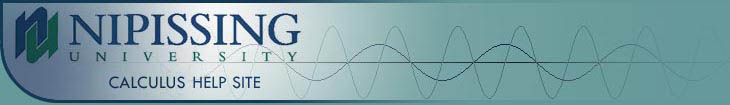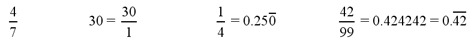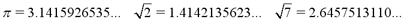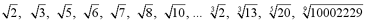TUTORIALS
TUTORIALS HOME

GENERAL MATH
NUMBER SETS
ABSOLUTE VALUE & INEQUALITIES
SETS & INTERVALS
FRACTIONS
POLYNOMIALS
LINEAR EQUATIONS
GEOMETRY
FINITE SERIES
TRIGONOMETRY
EXPONENTS
LOGARITHMS
INDUCTION

CALCULUS
LIMITS
DERIVATIVES
RELATED RATES & OPTIMIZATION
CURVE SKETCHING
INTEGRALS
AREA & VOLUME
INVERSE FUNCTIONS

MAIN
HOME
TESTS
TUTORIALS
SAMPLE PROBLEMS
COMMON MISTAKES
STUDY TIPS
GLOSSARY
CALCULUS APPLICATIONS
MATH HUMOUR

# Real Numbers

The set of real numbers is represented by the letter R. Every number (except complex numbers) is contained in the set of real numbers. When the general term "number" is used, it refers to a real number. All of the following types or numbers can also be thought of as real numbers.

# Integers

The set of integers is represented by the letter Z. An integer is any number in the infinite set,

Z = (..., -3, -2, -1, 0, 1, 2, 3, ...}

Integers are sometimes split into 3 subsets, Z+, Z- and 0. Z+ is the set of all positive integers (1, 2, 3, ...), while Z- is the set of all negative integers (..., -3, -2, -1). Zero is not included in either of these sets . Znonneg is the set of all positive integers including 0, while Znonpos is the set of all negative integers including 0.

# Natural Numbers

The set of natural numbers is represented by the letter N. This set is equivalent to the previously defined set, Z+. So a natural number is a positive integer.

N = { 1, 2, 3, 4, ... }

# Whole Numbers

The set of whole numbers is represented by the letter W. This set is equvalent to the previously defined set, Znonneg. So a whole number is a member of the set of positive integers (or natural numbers) or zero.

W = { 0, 1, 2, 3, 4, ... }

# Rational Numbers

The set of rational numbers is represented by the letter Q. A rational number is any number that can be written as a ratio of two integers. The set of rational numbers contains the set of integers since any integer can be written as a fraction with a denominator of 1. A rational number can have several different fractional representations. For example, 1/2 is equivalent to 2/4 or 132/264. In decimal representation, rational numbers take the form of repeating decimals. Some examples of rational numbers are:# Irrational Numbers

The set of irrational numbers is represented by the letter I. Any real number that is not rational is irrational. These are numbers that can be written as decimals, but not as fractions. They are non-repeating, non-terminating decimals. Some examples of irrational numbers are:Note: Any root that is not a perfect root is an irrational number. So any roots such as the following examples, are irrational.• | Proof that the square root of 2 is irrational

| Top of Page |

COURSE HOMEPAGES
MATH 1036
MATH 1037

FACULTY HOMEPAGES
Alex Karassev
Ted Chase